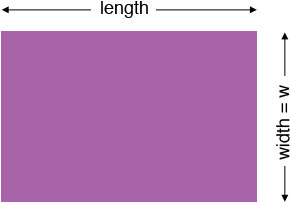SEARCH HOMEMath Central Quandaries & QueriesQuestion from jenna, a student: The length of a rectangular room is 2m more than the width. Find the dimensions if the perimeter of the room is 34mHi Jenna,

I drew a diagram and labeled the length and the width. I then let $w$ be the width of the room in meters.Write a mathematical expression for the length of the room using the variable $w.$ Now that you have an expression for the lengths of each of the four sides of the room write a mathematical expression for the perimeter of the room. You know that the perimeter is 34 meters so set your expression for the perimeter equal to 34 and solve for $w.$

PennyMath Central is supported by the University of Regina and The Pacific Institute for the Mathematical Sciences.